## Table of ContentOpen Access

ARTICLE

# Peak-Average-Power Ratio Techniques for 5G Waveforms Using D-SLM and D-PTS

Himanshu Sharma1, karthikeyan Rajagopal2, G. Gugapriya3, Rajneesh Pareek1, Arun Kumar4, Haya Mesfer Alshahrani5, Mohamed K. Nour6, Hany Mahgoub7, Mohamed Mousa8, Anwer Mustafa Hilal9,*

1 Department of Computer Science & Engineering, JECRC University, Jaipur, India
2 Centre for Nonlinear Systems, Chennai Institute of Technology, Chennai, India
3 School of Electronics Engineering, Vellore Institute of Technology, Chennai, India
4 Department of Electronics and Communication Engineering, JECRC University, Jaipur, India
5 Department of Information Systems, College of Computer and Information Sciences, Princess Nourah Bint Abdulrahman University, P. O. Box 84428, Riyadh, 11671, Saudi Arabia
6 Department of Computer Science, College of Computing and Information System, Umm Al-Qura University, Saudi Arabia
7 Department of Computer Science, College of Science & Art at Mahayil, King Khalid University, Saudi Arabia
8 Electrical Engineering Department, Faculty of Engineering and Technology, Future University in Egypt, New Cairo, 11845, Egypt
9 Department of Computer and Self Development, Preparatory Year Deanship, Prince Sattam bin Abdulaziz University, AlKharj, Saudi Arabia

* Corresponding Author: Anwer Mustafa Hilal. Email:Computer Systems Science and Engineering 2023, 45(2), 1199-1210. https://doi.org/10.32604/csse.2023.030909

## Abstract

Multicarrier Waveform (MCW) has several advantages and plays a very important role in cellular systems. Fifth generation (5G) MCW such as Non-Orthogonal Multiple Access (NOMA) and Filter Bank Multicarrier (FBMC) are thought to be important in 5G implementation. High Peak to Average Power Ratio (PAPR) is seen as a serious concern in MCW since it reduces the efficiency of amplifier use in the user devices. The paper presents a novel Divergence Selective Mapping (DSLM) and Divergence Partial Transmission Sequence (D-PTS) for 5G waveforms. It is seen that the proposed D-SLM and PTS lower PAPR with low computational complexity. The work highlighted a combination of multi-data block partial transmit schemes along with tone reservation. In this, an overlapping factor is used to determine the number of data blocks for every group. Here, considering only those data blocks that have minimum signal power, the use of DSLM and DPTS are required to eliminate the segment’s peaks. Simulation results reveal that the suggested hybrid technique proves to be better than the conventional PTS scheme. Furthermore, the power saving performance of FBMC and NOMA is compared with the Orthogonal Frequency Division Multiplexing (OFDM) waveform.

## Keywords

1  Introduction

•   We aim to investigate the PAPR reduction algorithms for the 5G waveform.

•   We proposed a novel hybrid algorithm for the 5G waveform.

2  Multicarrier Waveform Techniques

In MCWT, the data is transmitted by dividing a single carrier into a number of subcarriers. In the last several years, several waveform methods have been studied and concluded that PAPR is one of the big issues in multicarrier waveforms.

2.1 OFDM

The structure of the OFDM waveform is given in Fig. 1. PAPR is the result of IFFT utilisation in the transmitting part of the OFDM structure .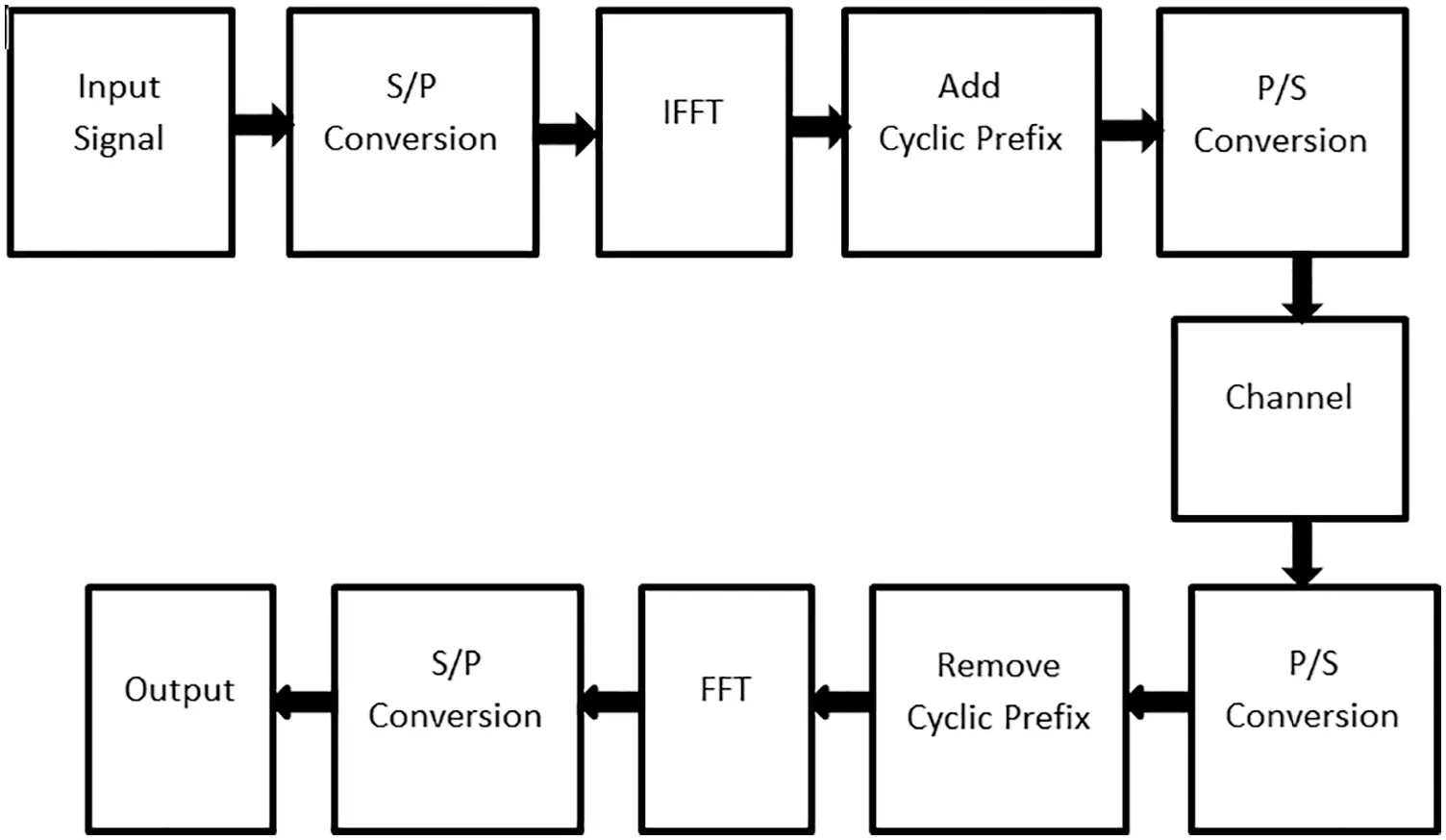Figure 1: OFDM schematic

The OFDM symbol can be represented as:

Y0={YL,L=0,1,,N1} (1)

where Yk is the OFDM symbol transmitted by Lth sub-carrier. The IFFT will convert the frequency domain OFDM signal into the time domain, given by:

y(n)=1Nl=0N1YLexpj(i2πLnN) (2)

Eq. (2) denotes the number of transmitted sub-carriers which increases the PAPR of the system. The PAPR of the OFDM signal can be written as:

PAPR(dB)=10log10[Max|yn|2E|yn|2] (3)

Here E is the expected operator.

2.2 FBMC

The structure of FBMC is shown in Fig. 2. A group of filters is used at the transmitting and receiving terminals of the FBMC scheme. A group of sub-carriers is filtered and processed to overcome the interference . The FBMC scheme does not add the Cyclic Prefix (CP). Hence, high PAPR is a common problem in OFDM and FBMC .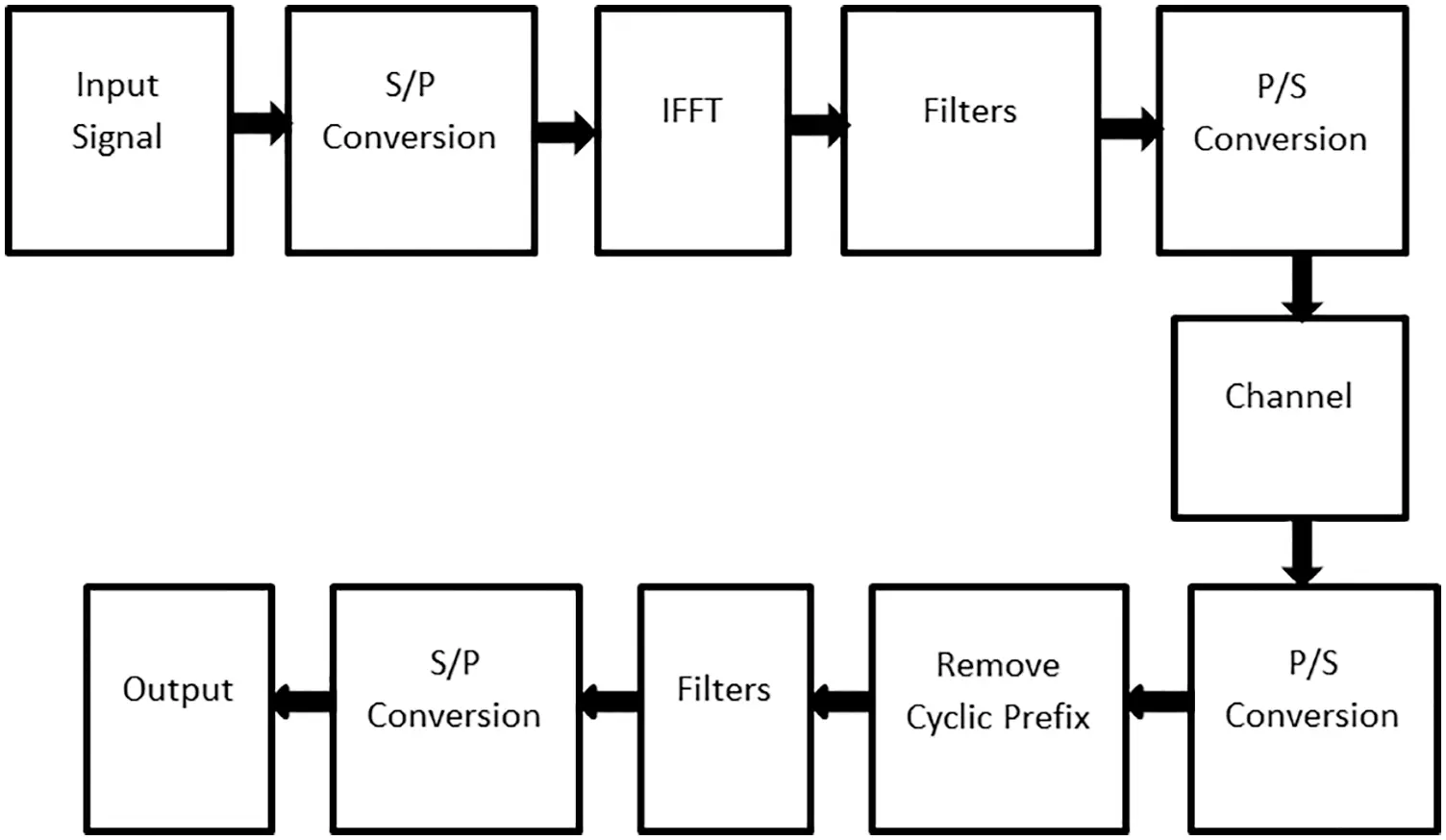Figure 2: FBMC schematic

The FBMC transmitted signal is given by:

YL=n=m=02N1Sm,nf(lnN)exp(iπm(ld2)N)exp(iθm,n) (4)

In this case yk is the FBMC symbol for L=0,1,,M1 . The number of sub-carriers is given by L = 2 N, Sm,n is the FBMC input signal at Lth sub-carrier, M, and n is the sub-carrier and symbol index, f(n) is the filter and d is the delay. In generally, θm,n is written as θm,n=3.142(m+n) and the filter g(n) is represented by:

f(n)=f(lin)eπM(Ld/2)N (5)

The PAPR of FBMC is given by:

PAPR(dB)=10log10Max|yL|2E|yL|2 (6)

The Complementary Cumulative Distribution Function (CCDF) is used to analyze the efficacy of PAPR algorithms, which is represented as:

CCDF=P(PAPR>Xt) (7)

where Xt is the threshold value.

2.3 NOMA

The schematic of the NOMA structure is given in Fig. 3. NOMA is attracting a lot of attention for 5G radio due to its several characteristics, such as low latency, high speed, and massive device connectivity. The transmitted NOMA signal is given by .

Yn=l=0N1Ynej2πnLN (8)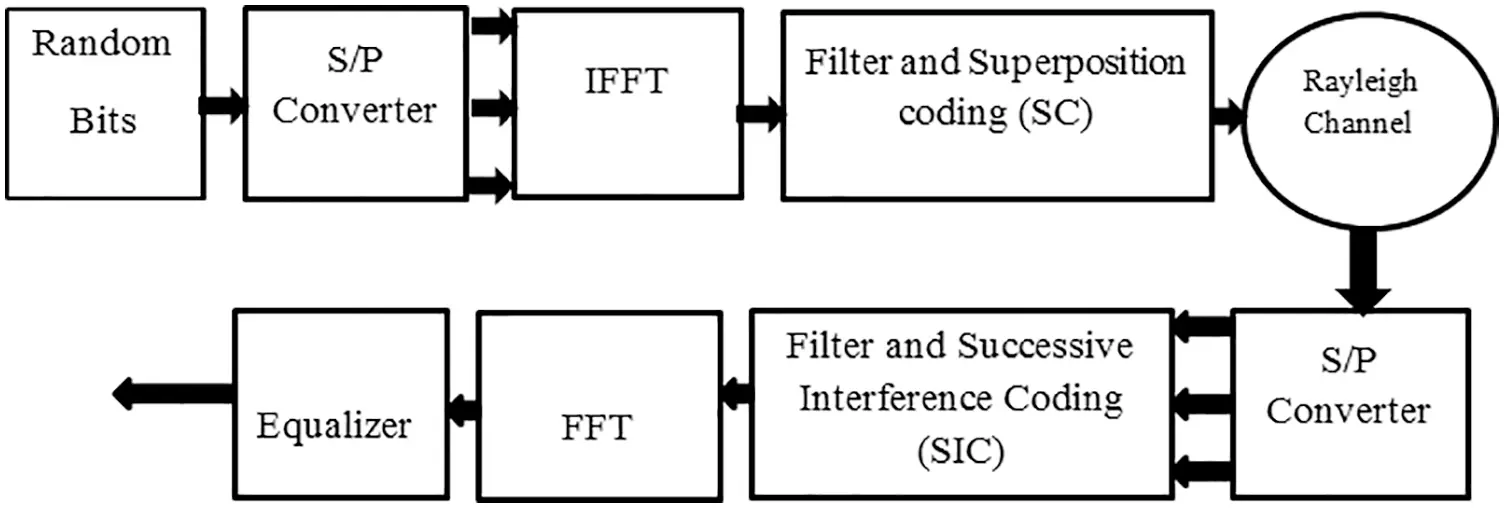Figure 3: NOMA

Considering Eq. (8), the PAPR is defined as:

PAPR(dB)=10log10[Max|yn|2E|yn|2] (9)

3  System Model

In recent years, there have been several PAPR algorithms investigated for OFDM and 5G waveforms.

3.1 D-SLM

Let us consider symbols mapped into the 64-QAM transmission scheme. Those symbols are loaded into parallel order, given as Sp . The series Sp symbols are divided into RSp(l) real and ISp(l) imaginary parts for l=0,1,,N1 . Thereafter, each element of a real and imaginary signal is multiplied with phase elements, pv(l)(0,1,,V1) where v represents the number of blocks and pv(l){1,1} . The Matrix Multiplication between real and imaginary signals by pv(l) is given by:

Svr=R{Sp}pv (10)

Svi=I{Sp}pv (11)

An IFFFT is applied to the Eqs. (10) and (11), given as:

yrv=IFFT(Prv) (12)

yiv=IFFT(Piv) (13)

Thereafter, the signal with the minimum PAPR value is transmitted, given by:

PAPR(dB)=10log10[Max|ynu|2E|ynu|2] (14)

where u represents the series of minimum PAPR values. The effectiveness of SLM is analysed by estimating CCDF, given as:

yv¯=yv={arg min0vv1(PAPRrvPAPRiv)} (15)

The Eq. (15) can be represented as:

Pr(PAPRv¯>PAPR0)=(1(1exp(PAPR0)αN)v2 (16)

where, α is define as PAPR reduction factor.

3.2 D-PTS

Let us consider a complex multicarrier signal for nth symbol given as:

{Yns,n=0,1,,Ns1,s=1,2,S} (17)

The Yns is divided in to v sub-blocks, given as:

Yvs=[Yv,0s,Yv,1s,,Yv,sNs1]T,0vV1 (18)

Hence, Yvs can be written as Yv=v=0V1Yvs . An IFFFT is applied at Yvs to estimate the time domain of the signal, given by:

yvs=IFFT(Yvs) (19)

To lower the PAPR, the time domain sequence Yvs is multiplied by phase rotation elements given as:

yv=v=0V1Pvuyv (20)

The Pvu is generated by using the Hadamard matrix given by:

Pvu(0vv1,0uu1) (21)

The ideal Pvu is estimated as:

Pv¯=argminPuPv(max0nN1|v=0V1Pvuyv,n|) (22)

4  Results

We have used Matlab-2014 to implement the PAPR algorithm techniques for multi-carrier waveforms. The key design factors are given in Tab. 1.4.1 PAPR Performance

In this section, we have analysed the efficiency of the D-PTS algorithm on NOMA, FBMC, and OFDM waveforms. It is seen that the D-PTS effectively reduces the PAPR of the waveforms and outperforms the conventional PTS method. Further, it is noted that by increasing the number of sub-blocks, the ideal PAPR value is obtained as Figs. 410 indicate that the D-PTS v = 16, w = 2 gave the best PAPR performance.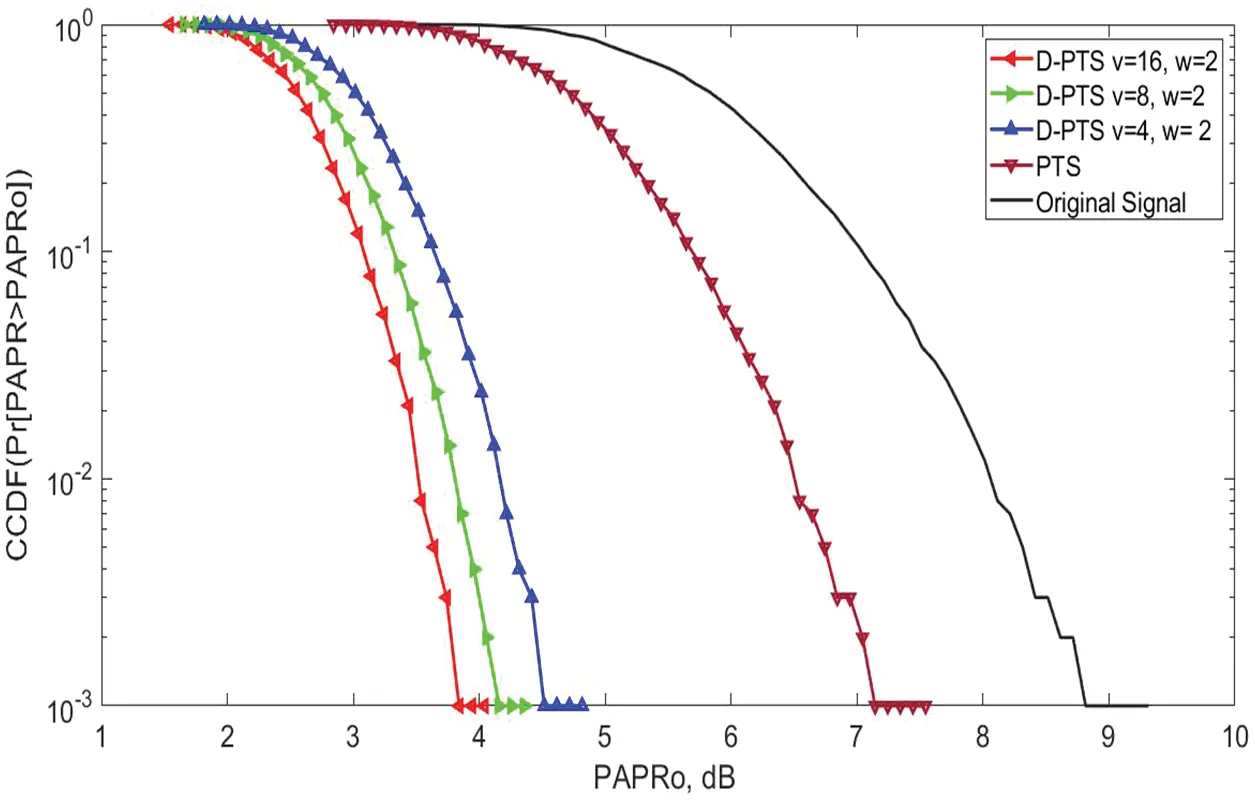Figure 4: PAPR vs. CCDF performance of the D-PTS and conventional PTS algorithms for the NOMA waveform using sub-blocks (u = 4, 8, and 16) and phase rotation elements (w = 2). The proposed D-PTS obtained a gain of 2.8 dB as compared with the PTS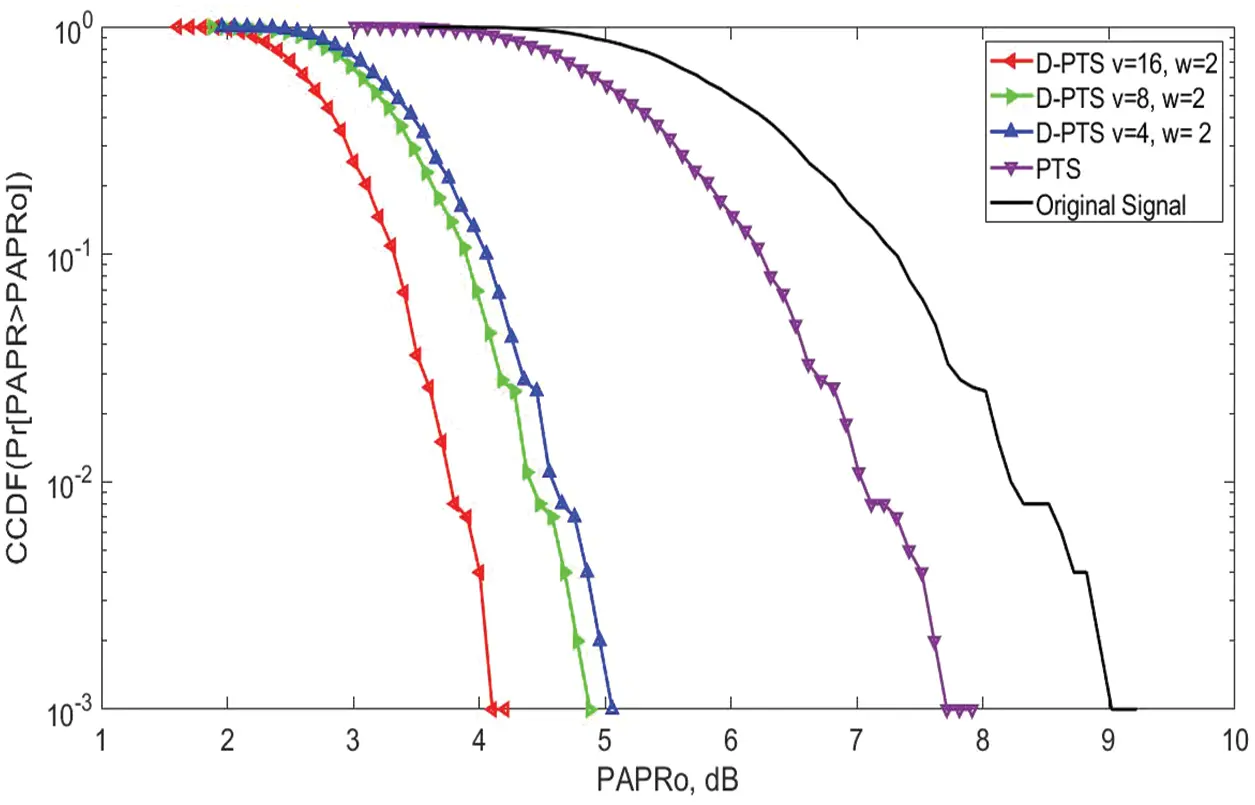Figure 5: At 10−3 CCDF-PAPR performance of the D-PTS and Conventional PTS algorithm for the FBMC waveform using sub-blocks (u = 4, 8 and 16) and phase rotation elements (w = 2). The proposed D-PTS obtained a gain of 3 dB as compared with the PTS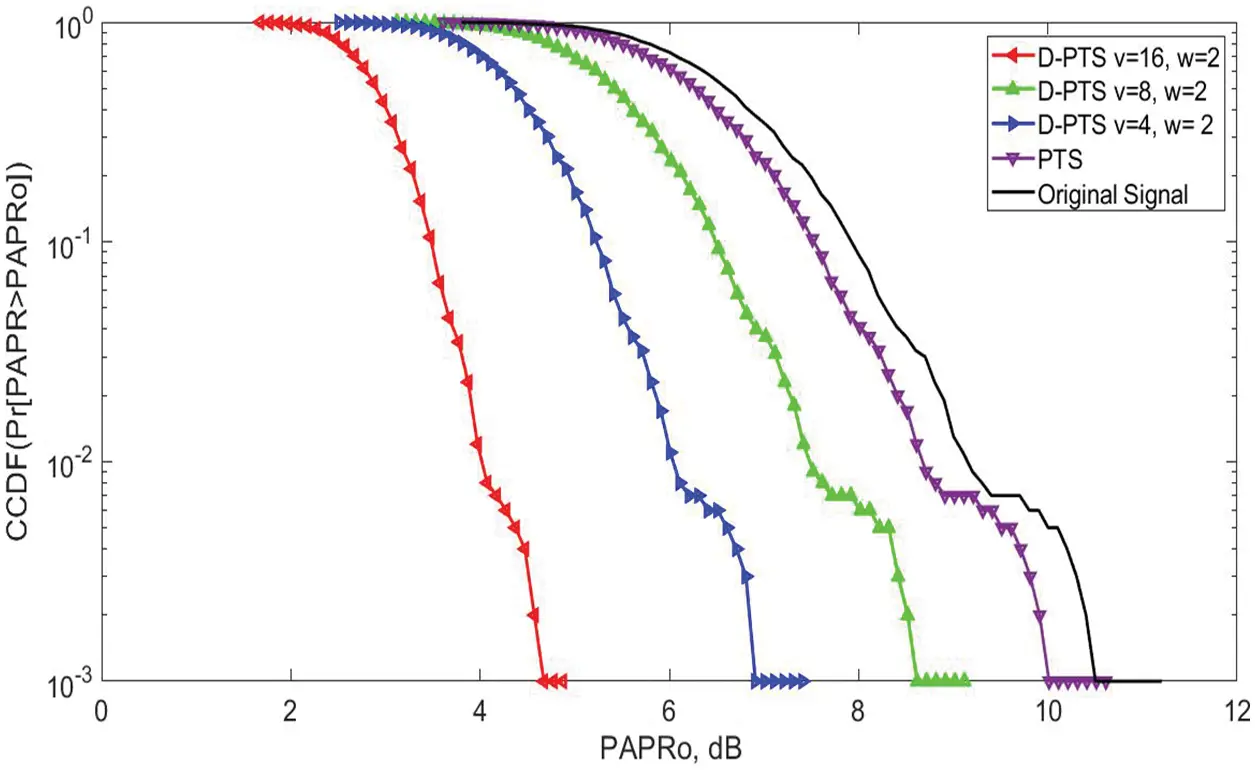Figure 6: PAPR vs. CCDF performance of the D-PTS and conventional PTS algorithms for the OFDM waveform using sub-blocks (u = 4, 8, and 16) and phase rotation elements (w = 2). The performance of the D-PTS is poor due to the difference in structural arrangement between the 5G and 4G waveforms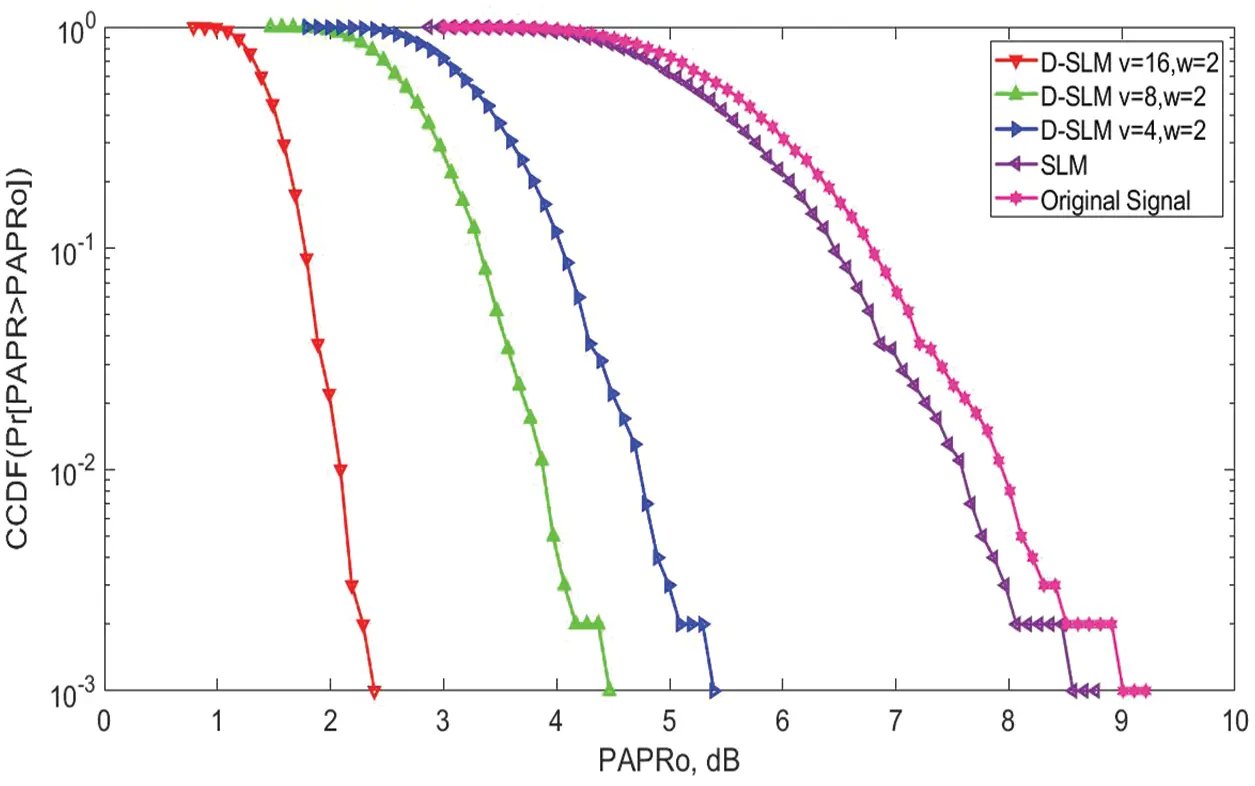Figure 7: PAPR vs. CCDF performance of the D-SLM and conventional SLM algorithms for the NOMA waveform using sub-blocks (u = 4, 8, and 16) and phase rotation elements (w = 2). The proposed D-SLM obtained a gain of 6.6 dB as compared with the PTS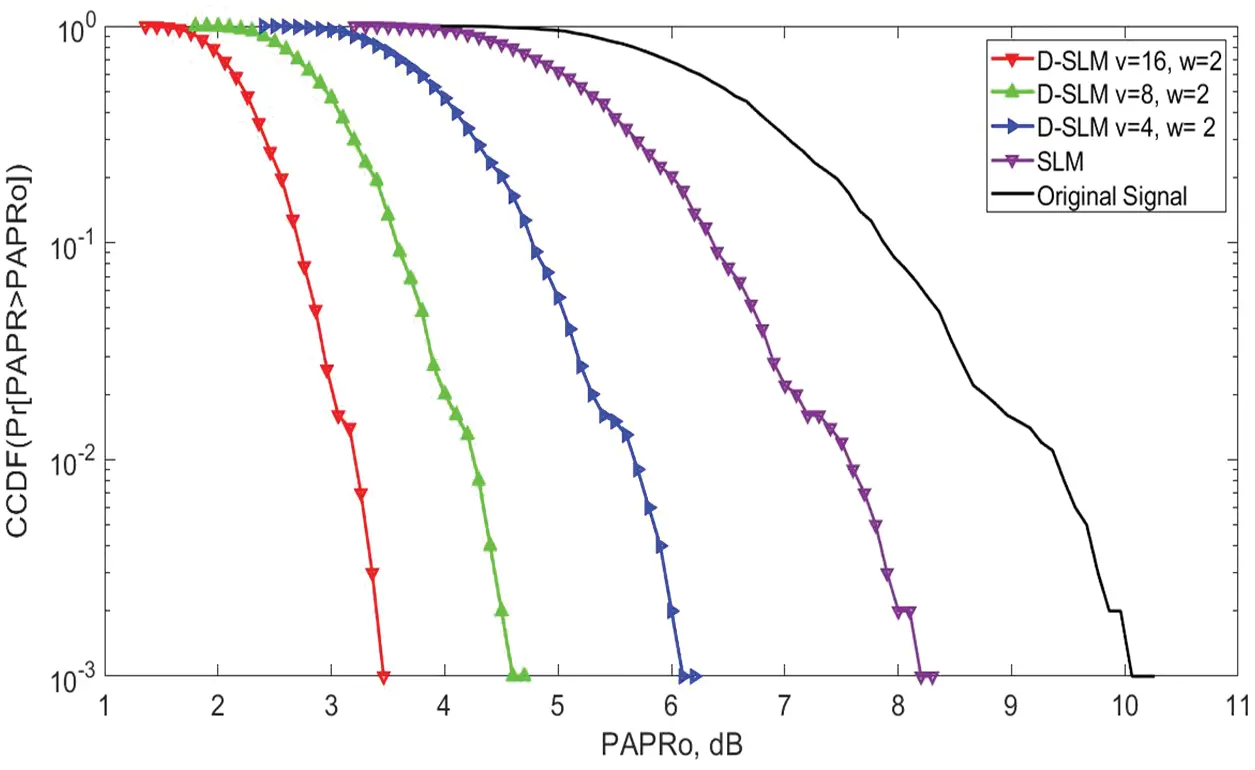Figure 8: At 10−3 CCDF-PAPR performance of the D-SLM and conventional PTS algorithm for the FBMC waveform using sub-blocks (u = 4, 8 and 16) and phase rotation elements (w = 2). The proposed D-SLM obtained a gain of 4.8 dB as compared with the PTS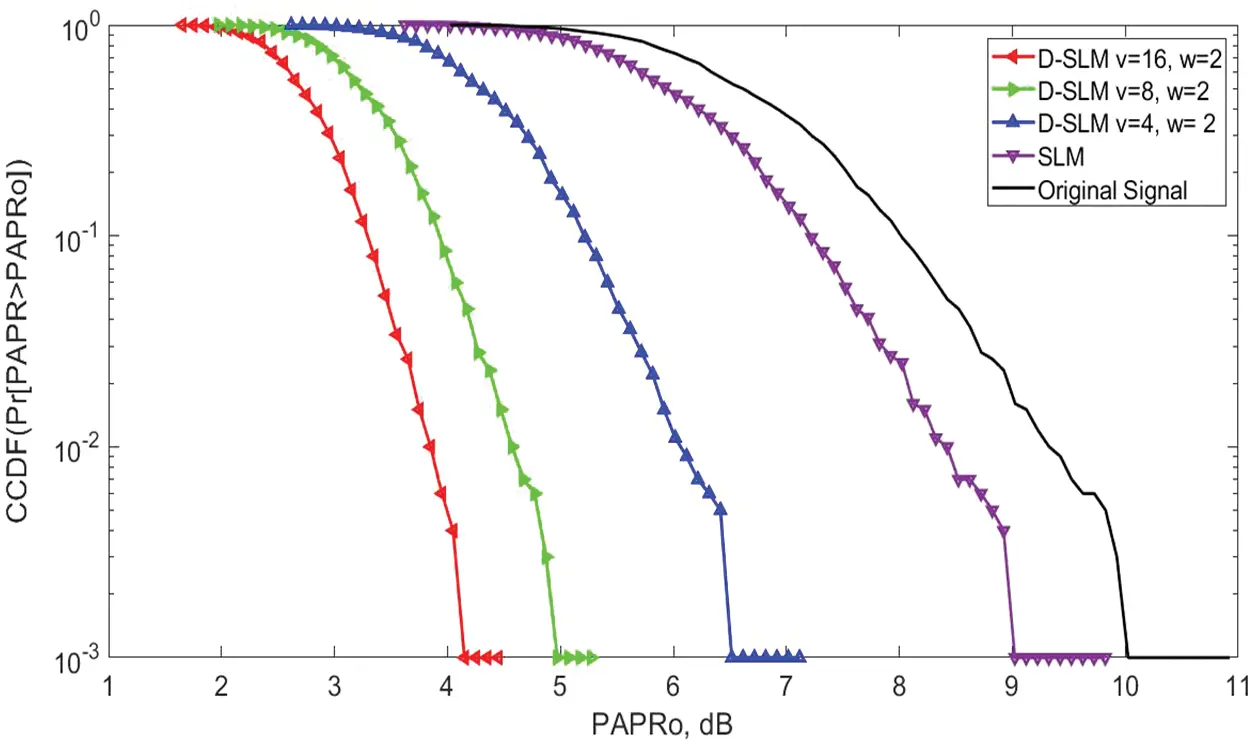Figure 9: PAPR vs. CCDF performance of the D-SLM and conventional PTS algorithms for the OFDM waveform using sub-blocks (u = 4, 8, and 16) and phase rotation elements (w = 2). The performance of the D-SLM is poor due to the difference in structural arrangement between the 5G and 4G waveforms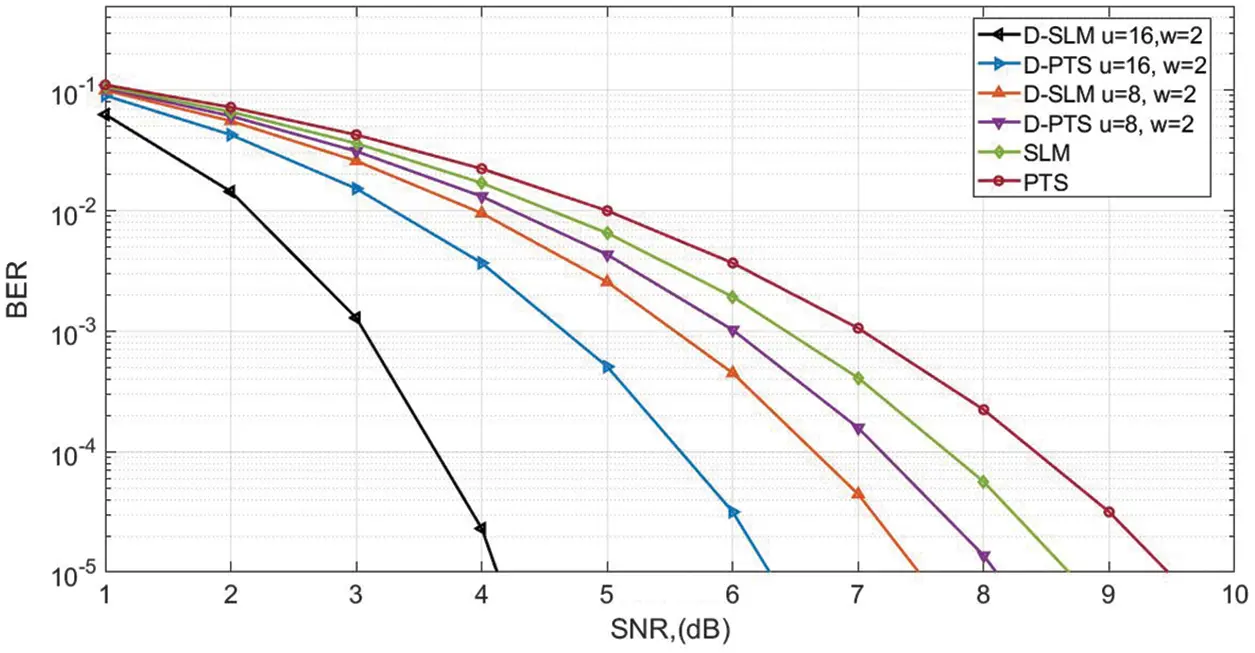Figure 10: SNR vs. BER

Finally, it is concluded that the proposed D-SLM gave an efficient PAPR performance and outperformed the D-PTS for the given waveforms.

4.2 BER Performance

In this part, we have studied the BER analysis of NOMA waveform after applying the proposed D-PTS and D-SLM algorithms to the Rayleigh channel. The proposed D-SLM, D-PTS (u = 16, 8 and w = 2) and conventional SLM and PTS achieved the BER of 10−5 at the SNRs of 4.2, 6.3, 7.5, 8.1, 8.7, and 9.2 dB as shown in Fig. 10. Hence, it is concluded that the D-SLM outperforms the other algorithms.

5  Conclusion

The work highlighted a combination of multi-data block DPTS and DSLM along with tone reservation. In this, an overlapping factor is used to determine the number of data blocks for every group. Here, considering only those data blocks that have minimum signal power, the use of a phase rotation element (w) is required to eliminate the segment’s peaks. Simulation results reveal that the suggested hybrid PTS-TR technique proves to be better than the conventional PTS scheme. The requirement of side information for each data block results in losses in data rates in selective mapping techniques. A novel D-SLM method has been proposed to reduce the complexity of the waveforms. The evaluation outcome shows that the suggested methods provide better performance in the reduction of PAPR and also promise a better BER.

Funding Statement: The authors extend their appreciation to the Deanship of Scientific Research at King Khalid University for funding this work under Grant Number (RGP 2/46/43). Princess Nourah bint Abdulrahman University Researchers Supporting Project Number (PNURSP2022R237), Princess Nourah bint Abdulrahman University, Riyadh, Saudi Arabia. The authors would like to thank the ‎Deanship of Scientific Research at Umm Al-Qura University ‎for supporting this work by Grant Code: 22UQU4310373DSR11.

Conflicts of Interest: The authors declare that they have no conflicts of interest to report regarding the present study.

## References

1. S. Sengupta and B. K. Lande, “An approach to PAPR reduction in OFDM using goppa codes,” Procedia Computer Science, vol. 167, pp. 1268–1280, 2020.
2. G. Monsalve, J. L. Ros, L. Brossier and J. M. Mestdagh, “An improved green ofdm scheme for papr reduction,” Telecom, vol. 1, pp. 196–210, 2020.
3. S. Thota, Y. Kamatham and C. S. Paidimarry, “Analysis of hybrid papr reduction methods of ofdm signal for hpa models in wireless communications,” IEEE Access, vol. 8, pp. 22780–22791, 2020.
4. R. Gopal and S. K. Patra, “Combining tone injection and companding techniques for papr reduction of fbmc-oqam system,” in 2015 Global Conf. on Communication Technologies (GCCT), Thuckalay, India, pp. 709–713, 2020.
5. F. Zou, Z. Liu, X. Hu and G. Wang, “A novel papr reduction scheme for ofdm systems based on neural networks,” Wireless Communications and Mobile Computing, vol. 2021, pp. 1–8, 2021. https://doi.org/10.1155/2021/5574807.
6. L. Dai, B. Wang, Z. Ding, Z. Wang, S. Chen et al., “A survey of non-orthogonal multiple access for 5 g,” IEEE Communications Surveys & Tutorials, vol. 20, no. 3, pp. 2294–2323, 2018.
7. P. P. Ann and R. Jose, “Comparison of papr reduction techniques in ofdm systems,” in 2016 Int. Conf. on Communication and Electronics Systems (ICCES), Coimbtore, INDIA, pp. 1–5, 2016.
8. R. Musabe, M. B. Lionel, M. Ushindi, M. Atupenda, J. Ntaganda et al., “PAPR reduction in LTE network using both peak windowing and clipping techniques,” Journal of Electrical Systems and Information Technology, vol. 6, no. 3, pp. 1–11, 2019. https://doi.org/10.1186/s43067-019-0004-1.
9. S. Sengar and P. P. Bhattacharya, “Performance improvement in ofdm system by papr reduction,” Signal & Image Processing: An International Journal (SIPIJ), vol. 3, no. 2, pp. 157–159, 2012.
10. S. Sarowa, N. Kumar and S. Agrawal, “Evolution of PAPR reduction techniques: A wavelet based OFDM approach,” Wireless Personal Communications, vol. 115, pp. 1565–1588, 2020.
11. M. M. Hasan, “A new papr reduction technique in ofdm systems using linear predictive coding,” Wireless Personal Communications, vol. 75, pp. 707–72, 2014.
12. N. V. Neut, B. T. Maharaj, F. H. Lange, G. González, F. Gregorio et al., “Papr reduction in fbmc systems using a smart gradient-project active constellation extension method,” in 2014 21st Int. Conf. on Telecommunications (ICT), Lisbon, Portugal, pp. 134–139, 2014.
13. I. A. Shaheena, A. Zekrya, F. Newagya and R. Ibrahimb, “Performance evaluation of papr reduction in fbmc system using nonlinear companding transform,” ICT Express, vol. 5, pp. 41–46, 2018.
14. L. Kaiming, H. Jundan, Z. Peng and L. Yuanan. “PAPR reduction for fbmc-oqam systems using p-pts scheme,” The Journal of China Universities of Posts and Telecommunications, vol. 22, no. 6, pp. 78–85, 2015.
15. N. A. Harthi, Z. Zhang, D. Kim and S. Choi, “Peak-to-average power ratio reduction method based on partial transmit sequence and discrete Fourier transform spreading,” Electronics, vol. 10, no. 6, pp. 642. 2021.
16. A. Kumar, M. Gupta, D. Le and A. A. Aly, “Pts-papr reduction technique for 5G advanced waveforms using BFO algorithm,” Intelligent Automation & Soft Computing, vol. 27, no. 3, pp. 713–722, 2021.
17. A. Kumar and H. Rathore, “Reduction of papr in fbmc system using different reduction techniques,” Journal of Optical Communications, vol. 42, no. 2, pp. 303–309, 2021.
18. S. Senhadji, Y. M. Bendimerad and F. T. Bendimerad, “Enhancing papr reduction for fbmc-oqam systems by joint both tone reservation and companding methods,” Indonesian Journal of Electrical Engineering and Computer Science, vol. 21, no. 2, pp. 919–926, 2021.
19. A. kumar and H. Rathore, “Modified dslm technique for papr reduction in fbmc system,” Radio Electronics and Communications Systems, vol. 62, no. 8, pp. 416–421, 20
20. M. Kazemian, J. Abouei and A. Anpalagan, “A low complexity enhanced-noma scheme to reduce inter-user interference, ber and papr in 5 g wireless systems,” Physical Communication, vol. 48, pp. 101–412, 2021.
21. M. K. Sharma and A. Kumar, “PAPR reduction in noma by using hybrid algorithms,” Computers, Materials & Continua, vol. 69, no. 1, pp. 1391–1406, 20
22. A. Kumar, “A novel hybrid papr reduction technique for noma and fbmc system and its impact in power amplifiers,” IETE Journal of Research, 2019. https://doi.org/10.1080/03772063.2019.1682692.
23. I. Baig, N. Hasan, M. Zghaibeh, I. U. Khan and A. S. Saand, “A dst precoding based uplink noma scheme for papr reduction in 5 g wireless network,” in 2017 7th Int. Conf. on Modeling, Simulation, and Applied Optimization (ICMSAO), Sharjah, United Arab Emirates, pp. 1–4, 2017.
24. T. Tao, Y. Mao and G. Hu, “A fair power allocation approach to OFDM-based NOMA with consideration of clipping,” Electronics, vol. 9, no. 10, pp. 1743, 2020.
25. A. Kumar and M. Gupta, “A comprehensive study of papr reduction techniques: Design of dslm-ct joint reduction technique for advanced waveform,” Soft Computing, vol. 24, pp. 11893–11907, 2020.
26. A. Kumar, M. A. Albreem, M. Gupta, M. H. Alsharif and S. Kim, “Future 5 g network based smart hospitals: Hybrid detection technique for latency improvement,” In IEEE Access, vol. 8, pp. 153240–153249, 2020.
27. A. Kumar, “Design and simulation of mimo and massive mimo for 5 g mobile communication system,” Int. Journal of Wireless Mobile Computing, vol. 14, no. 2, pp. 197–207, 2018.
28. A. Kumar, “Detection in 5G mobile communication system using hybrid technique,” National Academic Science Letter, vol. 44, pp. 39–42, 2021.
29. A. Kumar and M. Gupta, “A review on activities of fifth generation mobile communication system,” Alexandria Engineering Journal, vol. 57, pp. 1125–1135, 2018.

H. Sharma, K. Rajagopal, G. Gugapriya, R. Pareek, A. Kumar et al., "Peak-average-power ratio techniques for 5g waveforms using d-slm and d-pts," Computer Systems Science and Engineering, vol. 45, no.2, pp. 1199–1210, 2023.This work is licensed under a Creative Commons Attribution 4.0 International License , which permits unrestricted use, distribution, and reproduction in any medium, provided the original work is properly cited.

View

Like

## Related articles

• Neeraj Dubey, Geeta Agnihotri
• Dae-Young Kim, Se Dong Min, Seokhoon...
• Jalal Khan, Daniyal Ali Sehrai,...
• Yueping Wang, Xuan Zhang, Yixuan...
• Changming Zhao, Tiejun Wang, Alan...